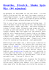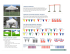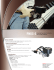# Measurements of string tension in a tennis racket R. Cross Abstract

## Transcription

Measurements of string tension in a tennis racket R. Cross Abstract
```Measurements of string tension in a tennis racket
R. Cross1 and R. Bower2
1
University of Sydney, Sydney, NSW 2006 Australia
University of Technology, Sydney, Australia
2
Abstract
The pull tension in a tennis string is always monitored while a racket is being strung, but
it is dif®cult to measure the string tension in a racket after it has been strung. In this
paper, a simple technique is described based on measurements of the vibration
frequency of the string plane. The key to this measurement is the fact that the vibration
frequency depends primarily on the area of the string plane and not its shape. It is shown
that there is a small loss in tension with time after a racket is strung but there is a large
decrease in tension during the stringing process. The tension immediately after stringing
is typically about 30% lower than the pull tension. Additional experiments are
described, showing that the large drop in tension is due to a combination of factors
including stress relaxation, frame distortion and friction between the strings.
Keywords: tennis, strings, tension, vibration frequency, elliptical membrane break
Introduction
One of the factors that determines the overall
performance of a tennis racket, and one that can
be selected by the player, is the string tension
(Groppel et al. 1987; Bower & Sinclair 1999;
Knudson 1997; Cross 2000). Most players have a
preferred tension of around 28 kg (274 N or about
62 lb) and some will even specify the tension to the
nearest 0.5 kg when they restring their racket. After
the racket is strung, the tension decreases slowly
with time as a result of stress relaxation, even if
the racket is not used. A recreational player will
typically get a restring every year or so, but
professionals usually restring their rackets prior to
every match in an attempt to ensure that the
tension is the same in each racquet and at the start
of every match.
It is not widely known that the tension in a tennis
string decreases rapidly during the stringing
Rod Cross, University of Sydney, Sydney, NSW 2006,
Australia. Fax: + 61 2 9351 7727.
E-mail: [email protected]
Ó 2001 Blackwell Science Ltd · Sports Engineering (2001) 4, 165±175
process. Thereafter, the tension decreases at a
steadily decreasing rate (Cross et al. 2000). One of
the reasons for the rapid drop in tension is the high
initial rate of stress relaxation. For example, if a
fresh sample of nylon string is tensioned to 28 kg
and then clamped at a ®xed length, the tension will
immediately start to decrease and will drop to about
24 kg within 15 min. Over the next 24 h, the
tension may drop by a further 1 or 2 kg, depending
on the type of string and its previous history.
Polyester strings lose tension more rapidly than
nylon, and a string that has previously been
stretched loses tension more slowly than one that
has not. String tension decreases with time due to
breakage of chemical bonds, which have a wide
distribution of strengths and which break gradually
over a period of time when the string is under
stress. The rate of breaking is largest when the
stress is ®rst applied. Once broken, bonds generally
remain broken, which explains why prestretching a
string reduces the subsequent rate of loss in tension.
During the stringing process, each string is
pulled to the desired tension before being clamped,
but once the tensioning head is disconnected, the
165
String tension in a tennis racket · R. Cross and R. Bower
tension drops rapidly. As each new string is added,
the racket frame pulls inwards slightly at points
on the frame that are not clamped, with the result
that the tension in the previous string is reduced.
With cheaper versions of stringing machines that
clamp the frame at only two points, and under a
total tension force of around 400 kg, the width of
the racket head can increase by up to 30 mm when
all the mains are strung. The racket head will then
pull back towards its original shape when the cross
strings are added, which acts to increase the tension
in each main string.
A third factor acting to reduce the tension during
the stringing process arises from friction between
the cross strings and the main strings. If a free
length of string is under stress, the tension at each
end is the same. However, when a cross string is
woven through the mains, the friction force acting
at each crossover point results in a decrease in
tension along the cross string. While one end may
be pulled at a tension of 28 kg, the other end may
be at a tension of only about 20 kg, depending on
the coef®cient of friction as well as the force
between the strings. The difference in tension
between one end of a cross string and the other
decreases after the racquet has been used to hit the
ball due to slippage or movement of the strings
across each other and through the grommet holes.
A fourth effect reducing the tension is that the
string is normally pulled at an angle of about
15 degrees to the string plane, so that the racket can
be rotated without hitting the pulling mechanism.
As a result of friction in the grommet holes, the
tension in the racket will be less than the pull
tension by 1 or 2 kg (i.e. about 7%).
During the stringing process one can measure the
pull tension at the free end of the string, but when
both ends of the string are tied to the racket frame,
the ®nal or subsequent string tension is not easily
determined. For this reason, string tension is
usually quoted in terms of the pull tension rather
than the tension at some later time of interest.
Various commercial devices have been developed to
monitor string tension after stringing, but the
accuracy of these devices is uncertain and the
resolution is generally poor. A good technique,
166
and one that is used in several commercial racket
diagnostic machines, is to apply a known force to
the centre of the string plane and to measure its
displacement. This provides a measure of string
plane stiffness, but the absolute value of the stiffness
or the string tension is not known since the
measured displacement depends not only on the
string tension but also on the length of each string,
the number of strings and the diameter of the object
used to apply the force. Nevertheless, this technique
can be used to monitor relative changes in string
tension over time in any given racket.
Another useful and simple tension measuring
device is sold under the trade name Stringmeter
(see http://www.stringmeter.com). It consists of
two cylindrical prongs separated by 8 mm which
are connected to a spring mechanism. The prongs
can be inserted anywhere in the string bed. The
prongs are rotated manually to exert a localized
sideways torque on a particular string, and the
tension is read off a calibrated dial. Provided that
one uses a consistent technique, and provided that
the string is relatively soft (e.g. natural gut or
nylon, but not kevlar) the local tension in any
particular string can be determined to within about
1 kg (Cross 2001a). Measurements with this device
indicate that the tension in the cross strings is
usually lower than the tension in the main strings.
Depending on the stringing machine and technique, the cross strings may even be 10 kg lower in
tension than the mains, even when all strings are
pulled at the same initial tension. Furthermore, the
tension can vary from one string to the next as well
as along a given string due to the effects of friction
in the grommet holes and between strings. The
friction forces are suf®ciently large that the tension
in the main strings usually remains signi®cantly
higher than the tension in the cross strings even
after several months of use.
Players themselves can obtain an indication of
string tension by hitting the strings and gauging the
frequency of the string vibrations by ear in the same
way that a musician can judge the tension in a
guitar or violin string. This technique allows a
player to compare one racket with another, but
the technique is limited by the fact that (a) the
Sports Engineering (2001) 4, 165±175 · Ó 2001 Blackwell Science Ltd
R. Cross and R. Bower · String tension in a tennis racket
vibration frequency also depends on the string mass
and length and (b) the frequency is not easily
committed to memory for future reference. Nevertheless, one can measure and record the vibration
frequency using appropriate instrumentation.
Commercial devices are available for this purpose,
such as the Tecni®bre ERT 700 or the Gamma
EST, which provide a digital reading of string
tension. The reading is obtained by attaching a
®xed mass to the strings (about 30 g) and then
driving the mass with an external oscillator or by
tapping the racquet frame to ®nd the resonance
frequency of the string-mass system. The conversion factor from frequency to tension has been
arti®cially chosen by the manufacturers so that the
indicated tension in a freshly strung racquet is close
to the pull tension. Two simple and inexpensive
techniques more suited for research purposes are
described in this paper and results are presented to
show that changes in string tension as small as 1%
can easily be measured. Both relative and absolute
changes in tension can be obtained by comparing
the measured frequencies with theoretical values.
Theoretical calculations presented below reveal
that the vibration frequency does not depend
signi®cantly on the shape of the racket head.
Consequently, the frequency can easily be calculated in terms of the string plane area, mass and
tension.
used by professional stringers as an industry
standard, but the conversion factor to string
tension is not known since the readings depend
on head size and number of strings. The DA
readings were, respectively, 55, 60, 63, 66 and 70 in
order of increasing tension, corresponding roughly
to the pull tension in lbs.
The string tension was monitored over a period
of nine days by measuring the vibration frequency
of the string plane. For this purpose, a small,
9 mm diameter, 0.5 mm thick piezo disk was
attached to the centre of the string plane using
blu-tack, which is a soft, pliable and re-usable
adhesive. The strings were set vibrating by tapping the racket frame with a ®nger and the voltage
output from the piezo was recorded on a storage
oscilloscope. The electrical connection from the
piezo to the oscilloscope was made with very ®ne
wires soldered to each electrode, together with a
voltage probe attached to the opposite ends of the
wire. A typical result is shown in Fig. 1. Without
the piezo in place, a faint but audible vibration of
the strings is heard, lasting typically one or two
seconds. When the piezo is attached to the
strings, the vibrations last for only about 0.1 s,
indicating that the blu-tack and piezo acts a string
dampener. However, a suf®cient number of vibration cycles were observed to enable the vibration
Experimental procedures
Five identical Volkl Pro Comp model graphite
rackets were strung with 16 mains and 19 crosses
using a 1.42 mm diameter Jadee brand nylon
string, at tensions 18.0, 21.0, 23.0, 25.2 and
28.4 kg. The head size was medium with a string
area 630 cm2 (98 in2). The fundamental vibration
frequency of each racket was measured to be
133  2 Hz, indicating a racket of medium stiffness.
The tension of the stringing machine was calibrated with a load cell immediately prior to stringing. The string plane stiffness was measured the
following day using a Paci®c racket diagnostic
machine calibrated in DA units. These units are
Ó 2001 Blackwell Science Ltd · Sports Engineering (2001) 4, 165±175
Figure 1 Output signal from a small piezo mounted in the
centre of the string bed in a tennis racket when the frame is
tapped.
167
String tension in a tennis racket · R. Cross and R. Bower
frequency to be determined to a precision of 0.2%
by measuring the time interval for 25 vibration
cycles.
The vibration frequency of the strings, without
the piezo in place, was also measured using a
microphone located close to the string plane. The
microphone was used to calibrate the effect of the
®nite piezo mass, but it was not used routinely since
the output signal from the microphone was only
about 0.5 mV. The piezo signal was much larger
(about 1 V) in amplitude and less `noisy', and it was
therefore more convenient to use. Nevertheless,
suitable electronic ampli®cation and ®ltering of a
microphone signal would provide an inherently
better measurement technique since it would
negate the necessity for further calibration and
correction. The combined mass of the piezo and
the blu-tack was 2.2 g, which is relatively small
compared with the 14.8 g mass of the strings, but it
lowered the vibration frequency of the string plane
by 15.7%, independent of the string tension. The
change in frequency due to the 2.2 g mass is not
signi®cant if one is interested only in relative
changes in string tension, provided that the combined mass of the piezo and adhesive is kept
constant for each measurement. However, a 15.7%
correction for the additional mass is quoted in all
results presented below.
As described in more detail below, the vibration
frequency
is given to a good approximation by
p
f  k T=m where T is the string tension, m is the
mass of the strings and k is a constant depending on
the number and length of the strings and the string
area. This expression is based on the formula for
the vibration frequency of a membrane clamped
around its edge or for a single string clamped at
each end. The fundamental vibration frequency
of the whole string plane is not the same as that
of a single string, but it is surprisingly close. The
decrease in frequency due to the addition of the
piezo depends on the point of attachment and is a
maximum at the centre of the string plane and a
minimum at points close to the racket frame. There
is no change in frequency at all if mass is added at a
vibration node, which is located around the perimeter of the frame for the fundamental mode. The
168
piezo was attached to the centre of the string plane
since the vibration amplitude of the fundamental
mode is a maximum at that point and since the
amplitude of several other signi®cant modes is a
minimum at that point.
Frequency measurements
Frequency measurements for all ®ve rackets
recorded over a period of 200 h are shown in
Fig. 2. All rackets were used by players in the
period from t  48 to t  53 h, and each racket
was used to hit about 300 forehands at medium
pace. The purpose was to determine how well
players could pick differences in string tension,
and the results will be described elsewhere. As
shown in Fig. 2, there was a slight drop in string
frequency and hence in string tension over this
period, but the decrease in tension as a result of
hitting the ball was too small to distinguish it
from the natural decrease in time over the period
of the measurement. The main signi®cance of
these results is that the relative differences in
tension between the various rackets were maintained closely during the period that the rackets
were used. However, interesting additional information can be obtained by comparing the
Figure 2 Change in string vibration frequency with time for ®ve
identical rackets strung at different tensions from 18.0 to
28.4 kg. Each racket was used to hit about 300 forehands at
medium pace over a 5-h period from t  48 to t  53 h.
Sports Engineering (2001) 4, 165±175 · Ó 2001 Blackwell Science Ltd
R. Cross and R. Bower · String tension in a tennis racket
observed frequencies with theoretical calculations
and with independent laboratory tests on short
samples of the string used in the rackets.
Tension loss and impact measurements
The loss in tension in the fully strung rackets was
compared with independent measurements of the
loss in tension in a short length of the string held
at a ®xed length between metal jaws. A 320 mm
length of the string was mounted in a metal frame
so that the string could be stretched and the tension
could be measured at one end with a load cell
(Cross et al. 2000). The string was tensioned to
either 18 or 28 kg, held at that tension for 10 s, and
then clamped at each end. The tension then
decreased with time as shown in Fig. 3. After
1000 s (16.7 min), the tensions dropped, respectively, by 2.14 kg (from 18.0 to 15.86 kg) and by
3.2 kg (from 28.0 to 24.8 kg). After three days
(72 h), the tensions dropped, respectively, from
18 to 14 kg and from 28.0 to 21.9 kg. After the ®rst
100 s, a plot of T vs. log(t) is clcosely linear.
Extrapolation of the data in Fig. 3 indicates that
the tension in the 28 kg string will drop to 19.1 kg
after one year. The rate at which T decreases is
approximately proportional to T. The implication
is that the two curves in Fig. 3 will eventually
Figure 3 Decrease in tension with time for strings initially
tensioned to 18 or 28 kg, and then clamped at a ®xed length in
a metal frame.
Ó 2001 Blackwell Science Ltd · Sports Engineering (2001) 4, 165±175
intersect, but the extrapolated intersection point is
at t  3.6 ´ 1015 years, at which time the tensions
will be close to zero.
The experiment was repeated for a shorter time
interval (1000 s), using fresh samples of the string,
after which the string was tested by impacting ten
times with a metal hammer mounted as a pendulum
as described by Cross et al. (2000). The hammer
impacted the centre of the string at right angles
with an impact energy of 1.63 J per impact,
equivalent in effect to about six very fast serves
each impact. The impact force was equivalent to
that for a single fast serve, but the impact duration
was about 30 ms, rather than the typical 5 ms
duration impact of a ball on the strings of a racket.
Each impact therefore had the equivalent effect on
loss in string tension of about six fast serves. By the
end of the ten impacts, the tension in the 18 kg
string dropped by an additional 0.74 kg and the
tension in the 28 kg string dropped by an additional 0.90 kg. About half of the drop occurred
during the ®rst impact. Subsequent impacts resulted in progressively smaller drops in tension.
Relation between frequency and tension
The tension at any time after stringing a racket can
be determined by comparing the observed string
vibration frequency with theoretical calculations of
the frequency. For this purpose, one can model the
string bed in most tennis racquets as an elastic
membrane, as described in the Appendix. The main
result is that the fundamental vibration frequency
of the string bed is given to a very good approximation by
s
1 T
1
f 
2L l
where T is the average string tension, l is the string
mass per unit length and
p L is the length of a single
string de®ned by L  A where A is the area of the
string bed. Some uncertainty regarding the area
arises if one considers the ®nite thickness of the
racket frame. The string plane has an `internal' and
an `external' area corresponding to the internal and
169
String tension in a tennis racket · R. Cross and R. Bower
external dimensions of the frame. The area of
interest extends to the end of each string which is
usually located near the outer edge of the frame.
A doubly surprising result is that (a) the vibration
frequency of the whole string bed is essentially the
same as that for a single string of length L and
(b) the vibration frequency is almost independent
of the shape of the string bed. Equation (1) agrees,
within 1%, with calculations for an elliptical
membrane, which is the conventional shape of the
string bed in most tennis rackets.
The string bed in the rackets described above
have an area A  630 cm2, so the equivalent single
string has length L  25.1 cm. Since the string used
in each racket had a mass density l  1.80 g m±1
(at tensions in the range
p 18±28 kg) the frequency
is given by f  145 T when T is expressed in
kg, or T (kg)  4.76 ´ 10±5f 2. Table 1 shows the
frequencies for each racket recorded immediately
after stringing, the pull tension Tp and the corresponding tensions after stringing, calculated from
the vibration frequencies.
The tensions immediately after stringing are all
about 30% lower than the pull tension. In each
case, the drop in tension is signi®cantly larger than
the drop due to stress relaxation. For example, at
the highest pull tension, stress relaxation over
15 min would account for a 3.2 kg drop in tension
in the ®rst string installed, but the drop in the last
string installed, about one minute prior to the
tension measurement, would be only about 1 kg.
Consequently, an additional experiment was conducted to measure string tension during the stringing process rather than immediately afterwards.
The experiment is described in the following
section.
Measurement of string tension using a load cell
In order to measure the tension in one of the cross
strings while stringing a racket, a load cell was
mounted in a metal frame attached externally to the
racket at the 3 o'clock position, as shown in Fig. 4.
The experiment was undertaken with a different
racket and a different string (1.30 mm diameter,
polyester) to those described above, but the results
highlight the fact that a signi®cant loss in tension
can arise from friction and from distortion of the
frame, even when the frame is securely clamped.
The racket was mounted in a Paci®c stringing
machine with clamps at six points around the
clamped internally at the 6 and 12 o'clock positions, which prevents motion radially inwards but
not outwards. It was clamped from above at the 2,
4, 8 and 10 o'clock locations, which prevents
radially outwards at those locations. A relatively
stiff polyester string was used for this experiment
since small changes in string length in a polyester
string result in much larger changes in string
Table 1 String tensions and frequencies
Tp (kg)
f (Hz)
T (kg)
Tp ) T
18.0
21.0
23.0
25.2
28.4
504
549
565
599
645
12.1
14.4
15.2
17.1
19.8
5.9
6.6
7.8
8.1
8.6
170
kg
kg
kg
kg
kg
Figure 4 Arrangement used to measure the tension in two cross
strings during and after the stringing of a tennis racket. The
main strings have been omitted for clarity.
Sports Engineering (2001) 4, 165±175 · Ó 2001 Blackwell Science Ltd
R. Cross and R. Bower · String tension in a tennis racket
tension than in a nylon string. Consequently, any
change in frame dimensions is more easily detected
with a polyester string than with a nylon string.
The tension indicator in the stringing machine
was ®rst calibrated against the load cell in order
to string the racket at a calibrated 28 kg. After
installing the main strings, the ®rst seven cross
strings were installed, and then the 8th and 9th
cross strings were threaded through a bracket
attached to the load cell and tensioned to 28 kg
as read by the tension indicator. The tension
indicated by the load cell was 51 kg (i.e. 25.5 kg on
average in each of Strings 8 and 9). Ideally, the load
cell would have indicated 56 kg (28 kg in each
string) but the alignment of the pull head, together
with friction in the grommet holes and friction
between the cross strings and the mains all acted to
reduce the average tension in Strings 8 and 9
to 25.5 kg. As each additional cross string was
partly to stress relaxation over time but mainly to
contraction of the frame. At the completion of the
stringing process and on removal of the racket from
the stringing machine, the load cell indicated
34.48 kg (17.24 kg per string). One hour later, it
indicated 33.8 kg (16.9 kg per string).
Immediately after stringing, the vibration frequency of the string bed was measured as 622 Hz.
The strung area was A  595 cm2 and the linear
mass density of the string at a tension of 28 kg was
l  1.73 g m±1. The mass density was measured at
zero tension, and then corrected for a measured
string extension of 3.8% at 28 kg. From eq. (1),
this indicates an average string tension of 16.9 kg.
A 1.2% correction to the frequency for an elliptical
shaped racket head gives T  16.6 kg. This is
slightly lower than, but consistent with, the tension
measured with the load cell, and much lower than
one would expect purely as a result of stress
relaxation. Measurements of tension loss in a short
sample of the string showed a decrease from 28 kg
to 23.3 kg over 1000 s (16.7 min).
No measurements were made of the tension in
the main strings, due to the restricted access, but
Love (2001) observed that the tension in the centre
main string drops as each new main string is added
Ó 2001 Blackwell Science Ltd · Sports Engineering (2001) 4, 165±175
and then increases as each new cross string is added.
The increase in tension arises partly as a result of
frame distortion and partly as a result of weaving the
cross strings, which acts to stretch or lengthen each
of the main strings typically by about 2 mm.
Discussion
Most racket stringers and tennis players are probably unaware that the string tension in a racket is
typically 30±40% lower than the speci®ed or pull
tension. Provided that the tension is lower by a
consistent amount, then the drop in tension during
the stringing process is of no real consequence to a
player, since players can specify whatever pull
tension they prefer. However, the drop in tension
could be of concern to a player if it is not a
consistent drop. The drop in tension is also of
signi®cance in a scienti®c sense if one wishes to
calculate or model the dynamic behaviour of a
racket using realistic racket and string parameters.
Examples of the latter type of calculation are the
®nite element models of a tennis racquet described
by Casolo & Lorenzi (2000) and by Winding &
Moeinzadeh (1990). The latter authors predicted
that the vibration frequency of a racquet frame
would increase when the strings are added. In fact,
the vibration frequency decreases (Cross 2001b).
Consistency in string tension requires considerable skill and patience on the part of the stringer, to
the extent that any interruption to the normal
routine (such as answering a telephone) could alter
the tension by 1 kg or more. It is probably not
possible to ensure that the tension is the same in
every string, nor is it necessary or even desirable.
For example, it is possible to reduce the stiffness of
the string plane near the edge of the racket frame if
one reduces the string tension or increases the
spacing between strings in the edge region. Furthermore, string tension by itself is not an important parameter in a tennis racket. The important
parameter is the string-bed stiffness, which depends
on factors such as head size and string type as well
as string tension. In this respect, the transverse
stiffness of the short cross strings will be closer to
the stiffness of the longer main strings if the tension
171
String tension in a tennis racket · R. Cross and R. Bower
in the cross strings is lower than the tension in the
main strings. An equally signi®cant parameter is
the ability of a player to distinguish between
different string tensions or different values of the
string-bed stiffness. If a player is unable to distinguish tension differences of say 10 kg (22 lb), then
the subtleties described in this paper would be of
no signi®cance to such a player.
Conclusions
In this paper, a relatively simple method has been
described by which the average string tension in a
racket can be determined after the racket is strung.
It was found that the tension immediately after
stringing with a nylon string is about 30% lower
than the pull tension, and the tension immediately
after stringing with a polyester string is about 40%
lower than the pull tension. It was also found that
the large loss in tension during the stringing
process can be attributed to four main factors,
namely (a) stress relaxation in a string under
tension (b) distortion of the racket head (c) friction
between the cross and main strings and (d) alignment of the tensioning head coupled with friction
between the string and the grommet holes.
Acknowledgements
The authors would like to thank Ron Kohn at Top
Serve Tennis, Burwood NSW for the loan of
rackets, the provision of string, technical assistance
References
Bower, R. & Sinclair, P. (1999) Tennis racket stiffness and
string tension effects on rebound velocity and angle for an
Appendix
Vibrations of the string bed can be described by
modelling it as a continuous membrane rather than
a discrete set of interconnected strings, in the same
way that one can describe vibrations of a so-called
continuous solid body with a discrete atomic
172
oblique impact. Journal of Human Movement Studies, 37,
271±286.
Brody, H. (1990) String bed oscillation formula. Stringer's
Assistant, 14(9), 18.
Casolo, F. & Lorenzi, V. (2000) On tennis equipment,
impact simulation and stroke precision. Tennis Science
and Technology. Blackwell Science, Oxford, UK,
pp. 83±90.
Cross, R. (1985) Demonstration of wave propagation in a
periodic structure. American Journal of Physics, 53,
563±567.
Cross, R. (2000) Flexible beam analysis of the effects of
string tension and frame stiffness on racket performance.
Sports Engineering, 3, 111±122.
Cross, R. (2001a) Measuring string tension. Racquet Tech,
July, 26, 14±18.
Cross, R. (2001b) Why bows get stiffer and racquets get
softer when the strings are added. American Journal of
Physics, 69, 907±910.
Cross, R., Lindsey, C. & Andruczyk, D. (2000) Laboratory testing of tennis strings. Sports Engineering, 3,
219±230.
Fletcher, N.H. & Rossing, T.D. (1991) The Physics of
Musical Instruments. Springer-Verlag, New York, NY,
USA.
Groppel, J.L., Shin, I.-S., Thomas, J.A. & Welk, G.J. (1987)
The effects of string type and tension on impact in
midsized and oversized tennis rackets. International
Journal of Sports Biomechanics, 3, 40±46.
Knudson, D. (1997) The effect of string tension on rebound
accuracy in tennis impacts. International Sports Journal, 1,
108±112.
Love, C. (2001) Frame distortion during stringing. Racquet
Tech, March 26, 16±18.
McLachlan, N.W. (1947) Theory and Application of
Mathieu Functions. Oxford University Press, London,
UK.
Winding, M.A.B. & Moeinzadeh, M.H. (1990) Finite
element modelling of a tennis racquet with variable string
patterns and tensions. International Journal of Sports
Biomechanics, 6, 78±91.
structure. Provided that the relevant wavelengths
are much larger than the spacing between the
strings, then the discrete structure of the membrane is irrelevant. This is the case for the
fundamental mode, where the wavelength is about
40 times larger than the spacing between strings.
The structure of the membrane may be signi®cant
Sports Engineering (2001) 4, 165±175 · Ó 2001 Blackwell Science Ltd
R. Cross and R. Bower · String tension in a tennis racket
if the spacing between strings is not constant, but it
is assumed below that the membrane is uniform.
The vibration frequency of a membrane, like that
of a single stretched string, is independent of the
elasticity or stiffness for a lengthwise stretch.
Provided that the vibration amplitude is small,
then the vibration frequency of a steel string is the
same as that of a nylon string of the same mass
density, the same length and at the same tension.
At any given tension, the vibration frequency of a
membrane depends primarily on its mass and
surface area and is almost independent of its shape.
For example, the fundamental vibration frequency
of a circular membrane of radius R and mass r per
unit area is given by (Fletcher & Rossing 1991)
r
2:405 F
2
f 
2pR
r
where F is the tension force per unit length acting
at right angles to any line drawn across the
membrane. For a rectangular membrane of sides
X and Y, the fundamental vibration frequency is
given by (Fletcher & Rossing 1991)
ÿ 2
1=2 r
X  Y2
F
3
f 
r
2XY
If the membrane is square, with sides X  Y  L
then
r
0:7071 F
4
f 
L
r
Suppose that a square membrane is constructed as a
grid of N strings in the x direction, N strings in the
y direction, all at the same tension T and with a
total mass M  2Nm where m is the mass of each
string. Then F  NT/L and r  M/L2, so
r
1 T
5
f 
2 mL
which is exactly the same as the fundamental
vibration frequency of a single, isolated string of
mass m, length L at tension T. This is to be
expected since all strings in a square grid would
vibrate in unison even if they were not connected
together, a result noted by Brody (1990). Note that
Ó 2001 Blackwell Science Ltd · Sports Engineering (2001) 4, 165±175
eq. (5) reduces to eq. (1) since m  lL. Note also
that the vibration frequency of the membrane
would be unaltered if the number of strings were
doubled, despite the fact that the mass of the string
bed and hence r would be twice as large. This is
because F would also double. If r is doubled simply
by doubling the mass of each string, without
increasing the number of strings, then thepvibration

frequency would decrease by a factor of 2.
For a rectangular membrane constructed from
strings in a square grid pattern, all at the same
tension, it is easy to show that the vibration
frequency of the whole membrane is close to the
average frequency of the individual long and short
strings. One would expect a similar result to hold if
the tensions in the long and short strings are
different, but this aspect of the problem was not
investigated in detail. The result will obviously
hold if the frequencies of the long and short strings
coincide, a condition which could well be approximated in some cases.
For each of the above cases, one can express the
frequency in terms of the surface area, A, of
the membrane. If fC, fS and fR are, respectively,
the fundamental frequencies for the circular, square
and rectangular membranes, then
fC  0:6784fo
6
fS  0:7071fo
7
and
8
fR  0:5r  1=r1=2 fo
p
where fo  F=rA and r  X/Y. Hence, fC/fS 
0.9594, and fR/fS  1.028 if (for example) r  1.4.
The frequencies for circular, square and rectangular membranes, all at the same tension and having
the same mass and surface area, differ by only a few
percent. One might therefore expect that the
frequency for an elliptical membrane will also be
similar, and that it might be slightly larger than that
of a circular membrane, given that the frequency
for a rectangular membrane is slightly larger than
that of a square membrane. This is indeed the case,
but there is no simple formula that one can quote
to describe an elliptical membrane. It is useful to
173
String tension in a tennis racket · R. Cross and R. Bower
Figure 5 Geometry of an elliptical membrane with semimajor
axis a, semiminor axis b and with foci at X   h. The
membrane boundary is at coordinate n  no.
note, however, that the wave velocity, v, for a
transverse wave in an elliptical membrane is the
samepas
that
 in any other membrane and is given by
v  F=r. It is also important to note that the
vibration frequency of a membrane depends on
both its mass and surface area. The quantity fo is
de®ned in terms of the membrane mass M  rA,
which appears to indicate that the frequency
depends only on the mass and not the surface area.
However, if M is ®xed and A is varied then F 
NT/L will also vary.
Solutions for an elliptical membrane can be
obtained if the wave equation for the membrane is
expressed in elliptical coordinates, in which case
the wave equation reduces to Mathieu's equation. If
the membrane is only slightly elliptical, then
analytical solutions can be found in terms of
Mathieu functions which can be expressed in the
form of an in®nite series. Unfortunately, the series
does not converge if the ratio of the major to the
minor axis is larger than about 1.1. A short
description of the Mathieu equation and its solutions is given by Cross (1985). A much more
extensive description, together with an analytical
treatment of the elliptical membrane problem, is
given by McLachlan (1947). An alternative solution
of the problem and one which is independent of the
shape of the ellipse, involves a direct numerical
solution of the wave equation. As shown by
McLachlan, the wave equation in elliptical coordi174
Fig. 6 Solutions of the wave equation for an elliptical mem-
brane with a/b  1.375 showing the membrane displacement as
a function of n and as a function g for the fundamental vibration
mode. The displacement amplitude is arbitrary but the amplitude is zero at the boundary where no  0.923, and it is the
same, for any given n, at g  0, p and 2p.
nates can be reduced to the two ordinary differential equations
d2 w
ÿ  p ÿ 2q cosh 2nw  0
dn2
9
and
d2 /
  p ÿ 2q cos 2g/  0
dg2
10
where (n, g) are the elliptical coordinates of any
point in the membrane, / describes the displacement of the membrane as a function of g, and
w describes the displacement as a function of n.
The displacement at any point is proportional
to both / and w but the amplitude is arbitrary.
The parameters p and q are de®ned in terms of the
semimajor axis a, the semiminor axis b and the
distance from the origin to the focus, h  (a2 ± b2)1/2,
as shown in Fig. 5. If the vibration frequency of the
membrane is expressed in the form
r
k1 F
11
fE 
2p r
then q  (k1 h/2)2. Equation (9) can be solved for
any assumed value of p to ®nd the value of q that
Sports Engineering (2001) 4, 165±175 · Ó 2001 Blackwell Science Ltd
R. Cross and R. Bower · String tension in a tennis racket
satis®es the boundary condition w(no)  0 where no
is the coordinate of the boundary of the membrane,
given by cosh(no)  a/h. This ensures that the
boundary remains at rest when the membrane
vibrates. The appropriate value of p must be found
by solving eq. (10) to ®nd a solution where / is
unchanged when g increases from 0 to 2 p. Equations
(9 and 10) can therefore be solved by iteration of
both p and q until a solution is found that satis®es
both boundary conditions. A typical solution is
shown in Fig. 6 for the rackets used in this experiment where a  0.165 m, b  0.120 m, a/b 
1.375, h  0.113 m, and no  0.923. For these
Ó 2001 Blackwell Science Ltd · Sports Engineering (2001) 4, 165±175
parameters, and for the fundamental vibration
mode, p  ±0.45, q  0.996, k1/2p  2.805 and
the area of the membrane is A  pab  622 cm2.
The vibration frequency in this case is 3%
larger than that of a circular membrane of the
same area.
Since fC  0.959fS and fE  1.03fC, then fE 
0.988fS. In other words, the vibration frequency
of the elliptical membrane is only 1.2% lower
than that of a square membrane of the same
area (A), and it is therefore only 1.2% lower than
the vibration
p frequency of a single string of
length L  A.
175
```

### Basic Information - Tecnológico de Monterrey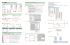### Flemish String Board Attachment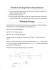### HOW TO CHANGE STRINGS ON A VB WITH THE HIPSHOT SYSTEM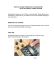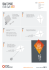### MiniRam 2T Front - New York Sewing Machines Inc.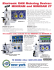### Zoë and her Zebra Mobile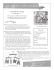### string playtest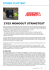### Breathe, Stretch, Shake Spin Mix (44 minutes)